Next: 2.2 Analytic MOSFET Approximations Up: 2. Simulation of Semiconductor Previous: 2. Simulation of Semiconductor

Subsections

# 2.1 Classical Semiconductor Device Equations

The semiconductor device equations can be used to describe the whole simulation domain of a semiconductor device. They are applied to the bulk semiconductor, the highly doped regions such as source and drain, and to dielectric regions such as the gate dielectric. In this section the classical semiconductor device equations are presented which are widely used for device simulation and their derivation.

## 2.1.1 Maxwell's Equations

The equations developed by James Clerk Maxwell describe the behavior of electric and magnetic fields and their interaction with matter. They were published by Maxwell in 1864  and in its original form comprised of 20 equations in 20 unknowns. Later they were reformulated in vector notation to the following form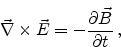(2.1)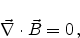(2.2)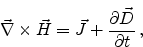(2.3)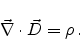(2.4)

Here,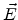is the electric field,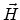the magnetic field,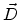the displacement vector, and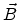the magnetic flux density vector.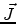denotes the conduction current density,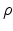is the electric charge density, and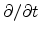is the partial derivative with respect to time.

Equation (2.1) expresses the generation of an electric field due to a changing magnetic field (Faraday's law of induction), (2.2) predicts the absence of magnetic monopoles (magnetic sources or sinks), (2.3) reflects how an electric current and the change in the electric field produce a magnetic field (Ampere-Maxwell law), and finally (2.4) correlates the creation of an electric field due to the presence of electric charges (Gauss' law).

We are using the Maxwell's equations to derive parts of the semiconductor device equations, namely the Poisson equation and the continuity equations.

## 2.1.2 Poisson's Equation

Poisson's equation correlates the electrostatic potential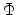to a given charge distribution. It can be derived from (2.4) using the relation between the electric displacement vector and the electric field vector,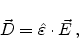(2.5)

whereis the permittivity tensor. This relation is valid for materials with time independent permittivity. As materials used in semiconductor devices normally do not show significant anisotropy of the permittivity,can be considered as a scalar quantity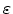in device simulation. The total permittivity is obtained from the relative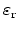and the vacuum permittivity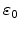as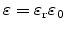. Table 2.1 gives an overview of relative permittivity constants for some materials commonly used in semiconductor devices.

Table 2.1: Relative permittivity constants for materials used in or considered for semiconductor devices.
 Material Relative Permittivity Si 11.7 GaAs 12.5 Ge 16.1 SiO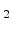3.9 HfO25 HfSiO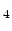15-18 ZrO20-25

As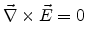for the stationary casecan be expressed as a gradient field of a scalar potential field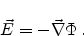(2.6)

Substituting (2.5) and (2.6) in (2.4) we get(2.7)

As we consider the permittivity a scalar, which is constant in homogeneous materials, we obtain Poisson's equation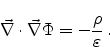(2.8)

The space charge densityconsists of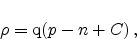(2.9)

where q is the elementary charge,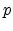and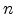the hole and electron concentrations, respectively, and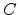the concentration of additional, typically fixed, charges. These fixed charges can originate from charged impurities of donor (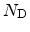) and acceptor (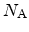) type and from trapped holes () and electrons (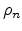),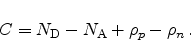(2.10)

The inclusion of trapped carriers is important for the simulation of the impact of degradation on the device performance (Section 2.2, Section 5.3.2, Chapter 6).

Together (2.8) and (2.9) lead to the form of Poisson's equation commonly used for semiconductor device simulation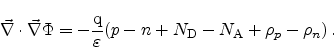(2.11)

## 2.1.3 Continuity Equations

The continuity equations can be derived from (2.3) by applying the divergence operator,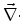, to the equation and considering that the divergence of the curl of any vector field equals zero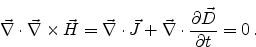(2.12)

Separating the total current densityinto hole and electron current densities,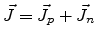, and using (2.4) and (2.9) gives(2.13)

When we consider the charged impurities as time invariant and introduce a quantity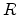to split up (2.13) into separate equations for electrons and holes, we get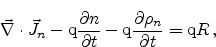(2.14)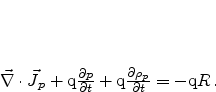(2.15)

The quantitygives the net recombination rate for electrons and holes. A positive value means recombination, a negative value means generation of carriers. Models forare presented in Section 2.3.

## 2.1.4 Current Relations

There are two major effects which lead to current flow in silicon. First, the drift of charged carriers due to the influence of an electric field, and second, the diffusion current due to a concentration gradient of the carriers.

### 2.1.4.1 Carrier Drift

Charged carriers in a semiconductor subjected to an electric field are accelerated and acquire a certain drift velocity. The orientation depends on the charge state, holes are accelerated in direction of the electric field and electrons in opposite direction. The magnitude of the drift velocity depends on the statistical probability of scattering events. At low impurity concentration, the carriers mainly collide with the crystal lattice. Is the impurity concentration higher the collision probability with the charged dopants through Coulomb interaction becomes more and more likely, thus reducing the drift velocity with increasing doping concentration.

For low electric fields, the drift component of the electric current can be expressed in terms of Ohm's law as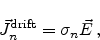(2.16)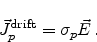(2.17)

Here,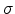denotes the conductivity and can be expressed in terms of carrier mobilities for electrons and holes,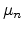and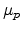, as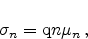(2.18)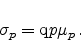(2.19)

The mobility for electrons is, due to their lower effective mass, about three times higher than for holes.

### 2.1.4.2 Carrier Diffusion

A concentration gradient of carriers leads to carrier diffusion. This is because of their random thermal motion which is more probable in the direction of the lower concentration. The current contribution due to the concentration gradient is written as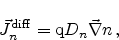(2.20)(2.21)

Here,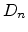and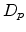are the diffusion coefficients for electrons and holes, which can, in thermal equilibrium for non-degenerate semiconductors, be expressed in terms of the mobility using the Einstein relation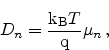(2.22)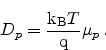(2.23)

### 2.1.4.3 Drift-Diffusion Current Relations

Combining the current contributions of the drift and the diffusion effect we get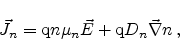(2.24)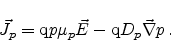(2.25)

## 2.1.5 The Semiconductor Equations

With the Poisson equation (2.11), the continuity equations for electrons and holes (2.14) (2.15), and the drift-diffusion current relations for electron- and hole-current (2.24) (2.25) we now have a complete set of equations which can be seen as fundamental for the simulation of semiconductor devices: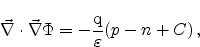(2.26)(2.27)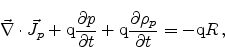(2.28)(2.29)(2.30)

These equations, not including the charge contribution of trapped carriers, have first been published by VanRoosbroeck in 1950 .

This set of equations is widely used in numerical device simulators and provides only the basics for device simulation. In modern simulators they are accompanied by higher order current relation equations like the hydrodynamic, six-, or eight-moments models. There are models for the carrier mobility, the carrier generation and recombination (Section 2.3), for quantum effects like quantum mechanical tunneling (Section 5.3) or quantum confinement (Section 2.4.1) and of course for modeling of device degradation, as negative bias temperature instability (Chapter 6).Next: 2.2 Analytic MOSFET Approximations Up: 2. Simulation of Semiconductor Previous: 2. Simulation of Semiconductor

R. Entner: Modeling and Simulation of Negative Bias Temperature Instability